A 10 kg mass moves along x-axis. Its acceleration as a function of its position is shown in the figure. What is the total work done on the mass by the force as the mass moves from x=0 to x=8 cm [This question is only for Dropper and XII batch]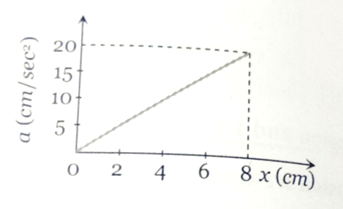1.

2.

3.

4.

Concept Questions :-

Integration
High Yielding Test Series + Question Bank - NEET 2020

Difficulty Level:

The relationship between force and position is shown in the figure given (in one dimensional case). Find the work done by the force in displaying a body from x= 1 cm to x= 5cm is   [This question is only for Dropper and XII batch]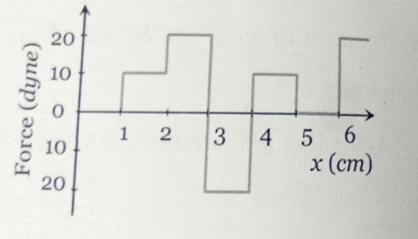1. 10 erg

2. 20 erg

3. 30 erg

4. 40 erg

Concept Questions :-

Integration
High Yielding Test Series + Question Bank - NEET 2020

Difficulty Level:

The graph between the resistive force F acting on a body and the distance covered by the body is shown in the figure. The mass of the body is 25 kg and initial velocity is 2 m/s. When the distance covered by the body is 4 m, its kinetic energy would be    [This question is only for Dropper and XII batch]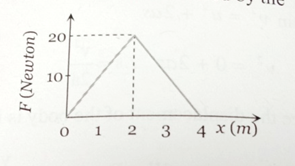1. 50 J

2. 40 J

3. 20 J

4. 10 J

Concept Questions :-

Integration
High Yielding Test Series + Question Bank - NEET 2020

Difficulty Level:

A particle which is constrained to move along the x-axis, is subjected to a force in the same direction which varies with the distance x of the particle from the origin as . Here k and a are positive constants. For $x\ge 0$, the functional form of the potential energy ${U}_{\left(x\right)}$ of the particle is

[This question is only for Dropper and XII batch]

1.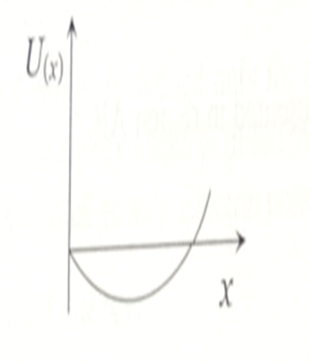2.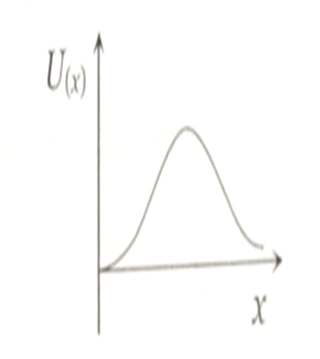3.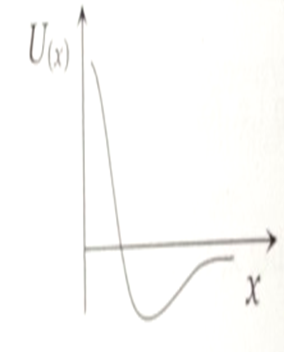4.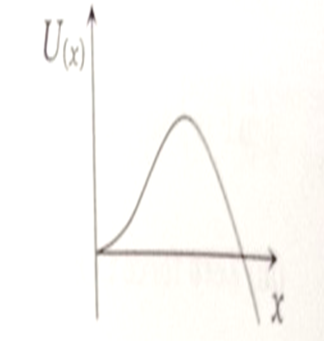Concept Questions :-

Integration
High Yielding Test Series + Question Bank - NEET 2020

Difficulty Level:

A long spring is stretched by 2 cm, its potential energy is U. If the spring is streched by 10 cm, find the potential energy stored in it.   [This question is only for Dropper and XII batch]

1. 10 U

2. 15 U

3. 20 U

4. 25 U

Concept Questions :-

Integration
High Yielding Test Series + Question Bank - NEET 2020

Difficulty Level:

A spring of spring constant  is stretched initially by 5 cm from the unstretched position. Find the work required to stretch it further by another 5 cm is   [This question is only for Dropper and XII batch]

1. 15 J

2. 18.75 N .m

3. 20 J

4. 22.75 N .m

Concept Questions :-

Integration
High Yielding Test Series + Question Bank - NEET 2020

Difficulty Level:

An automobile of mass m accelerates, starting from rest, while the engine supplies constant power P, its position and velocity changes w.r.t time as-    [This question is only for Dropper and XII batch]

1.

2.

3.

4.

Concept Questions :-

Integration
High Yielding Test Series + Question Bank - NEET 2020

Difficulty Level:

A constant force F is applied on a body. The power (P) generated is related to the time elapsed (t) as

[This question is only for Dropper and XII batch]

1.

2.

3.

4.

Concept Questions :-

Integration
High Yielding Test Series + Question Bank - NEET 2020

Difficulty Level:

A rod of length L is placed along the x-axis between x= 0 and x= L. The linear density (mass/length)$\lambda$ of the rod varies with the distance x from the origin as $\lambda$= Rx. Here, R is a positive constant. Find the position of centre of mass of this rod.

[This question is only for Dropper and XII batch]

1.

2.

3.

4.

Concept Questions :-

Integration
High Yielding Test Series + Question Bank - NEET 2020

Difficulty Level:

A wheel rotates with an angular acceleration . Here t is the time and a, b are constants. If the wheel has an initial angular velocity ${\omega }_{0}$, find (a) the angular velocity and (b) the angle turned as function of time

[This question is only for Dropper and XII batch]

Concept Questions :-

Integration